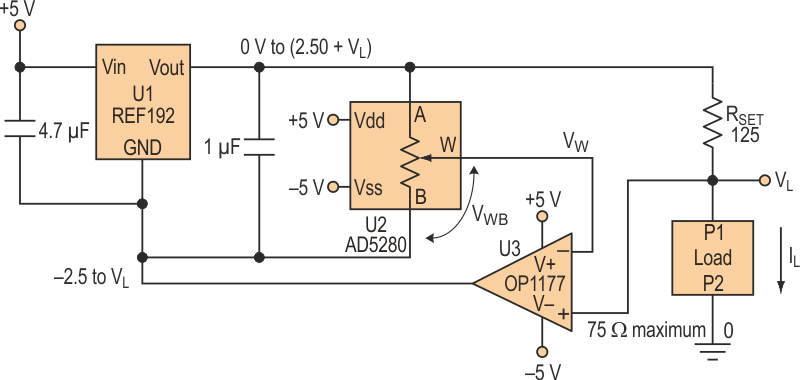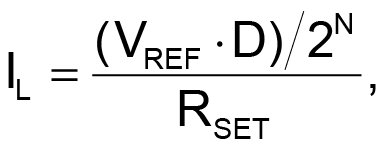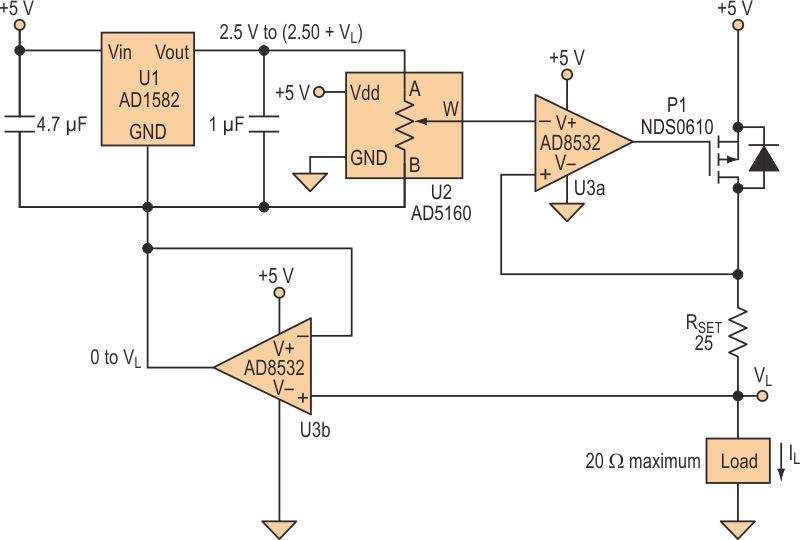# Precision Programmable Current Sources Use Digital Pots

Peter Khairolomour

Electronic Design

The circuit that's shown in Figure 1 implements a programmable 0- to 20-mA precision current source. The REF192 low-headroom 2.5-V voltage reference (U1) can source up to 30 mA. An AD5280 digital potentiometer (U2) controls the voltage-divider ratio of the reference voltage. U3, an OP1177 op amp, closes the loop by forcing VL = VW.Figure 1. This precision 0- to 20-mA programmable current source uses a digital potentiometer (U2) to control the voltage-divider ratio of the reference voltage.

At the digital pot's zero scale, where VWB ≈ 0 V, the voltage across RSET will approach zero and no current will flow through the load. The reference output also will approach 0 V, forcing the GND node to –2.5 V. At the digital pot's full scale, the GND node will be forced to VL and the reference output will thus be set to 2.50 V + VL. Dividing the voltage across terminals B-to-W (VREF × D/2N) by RSET can determine the general equation for the load current. RSET determines the range of achievable current(1)

where VREF = REF192 rated reference voltage, D = decimal equivalent of the AD5280 input code, and N = resolution of the AD5280.

For best power efficiency, choose the lowest RSET and the lowest VREF that has adequate output-current capability to achieve the desired current.

With RSET = 124.03 Ω and an RLOAD of 24.85 Ω, 51.093 Ω, and 75.05 Ω, actual laboratory tests of the output current versus the 8-bit digital pot setting show close conformance to the ideal output as predicted by the equation (see the equation). Users should pay attention to the load impedance because VL increases with the load, and it can't be driven beyond the op amp's rail. VL also determines the voltage on the REF192's GND pin, thereby limiting the operating headroom.

The single-supply precision 0- to 100-mA programmable current source that's shown in Figure 2 can be used in applications such as laser diode drivers and tunable lasers that require a boosted current without compromising precision. With this circuit, the entire system can operate from a single +5-V supply, as opposed to the ±5-V supply required for the previous circuit.Figure 2. Suitable for applications like laser diode drivers, this single-supply precision programmable current source features an output of 0 to 100 mA.

This advantage comes from the voltage swing at the ground pin of the AD1582 voltage reference being now strictly positive. As a result, the AD5160 single-supply digital potentiometer and AD8532 single-supply op amps are employed.

The same general current equation (see the equation, again) applies to this circuit. With RSET = 24.82 Ω, and an RLOAD of 5.185 Ω, 14.946 Ω, and 19.97 Ω, actual laboratory tests of the output current versus the 8-bit digital pot setting show close conformance to the ideal output, again as predicted by the above equation (see the equation, again).

It's important to note that a PMOS FET was used rather than an NMOS FET. If an NMOS FET were employed, the FET's source voltage would rise as the current increased, leading to a decrease in VGS. This would limit the drain current, defeating the purpose of the circuit. The PMOS FET isn't prone to this problem because the source is tied to VDD.

At first glance, the arrangement of the PMOS FET and AD8532 may seem like a positive feedback system. However, the PMOS placed in the feedback loop adds an extra inversion, providing overall negative feedback in the loop. Finally, the 100-kW version of the AD5160 was used to reduce the error voltage that arises from the voltage divider between RAB and the non-ideal wiper resistance of the digital potentiometer.

## Materials on the topic

electronicdesign.com